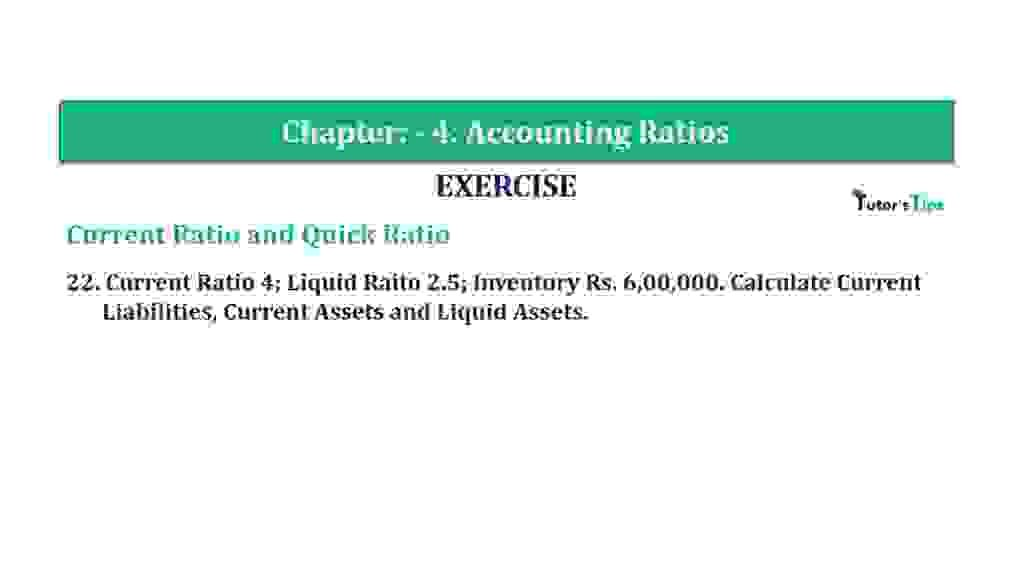# Question 22 Chapter 4 of +2-B – T.S. Grewal 12 ClassQuestion 22 Chapter 4 of +2-B

Current Ratio and Quick Ratio

22. Current Ratio 4; Liquid Raito 2.5; Inventory Rs. 6,00,000. Calculate Current Liabilities, Current Assets and Liquid Assets.

The Content covered in this article:

### The solution of Question 22 Chapter 4 of +2-B: –

 Current Ratio = Current Assets = 4 Current Liabilities 1

 Quick Ratio = Quick Assets = 2.5 Current Liabilities 1

 Inventories = 6,00,000 Let Current Liabilities = x Current Assets = 4x Liquid Assets = 2.5x Inventories = Current Assets – Quick Assets Rs. 6,00,000 = 4x – 2.5x Rs. 6,00,000 = 1.5x x = Rs. 4,00,000 Current Liabilities = x = Rs. 4,00,000

 Current Assets = 4 x 4,00,000 = Rs. 16,00,000

 Liquid Assets = 2.5 x Rs. 4,00,000 = Rs. 10,00,00

Balance Sheet: Meaning, Format & Examples

Thanks, Please Like and share with your friends

Comment if you have any question.

Also, Check out the solved question of previous Chapters: –

### T.S. Grewal’s Double Entry Book Keeping (Vol. II: Accounting for Companies)T.S. Grewal’s Analysis of Financial Statements